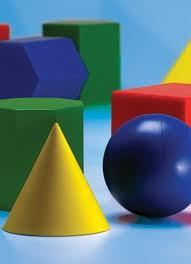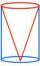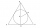# Cone in cube

The cube is inscribed cone. Determine the ratio of the volume of cone and cube.

The ratio express as a decimal number and as percentage.

r =  0.26
r =  26.2 %

### Step-by-step explanation:

$r=\frac{\pi }{12}=0.26$
$r=100\cdot \frac{\pi }{12}=26.2\mathrm{%}$Did you find an error or inaccuracy? Feel free to write us. Thank you!Tips to related online calculators
Tip: Our volume units converter will help you with the conversion of volume units.

## Related math problems and questions:

• Cone in cylinderThe cylinder is inscribed cone. Determine the ratio of the volume of cone and cylinder. The ratio express as a decimal number and as percentage.
• InscribedCube is inscribed in the cube. Determine its volume if the edge of the cube is 10 cm long.
• Inscribed sphereHow many percent of the cube volume takes the sphere inscribed into it?
• LatheFrom the cube of edge, 37 cm was a lathed maximum cylinder. What percentage of the cube is left as waste after lathed?
• Cube into sphereThe cube has brushed a sphere as large as possible. Determine how much percent was the waste.
• Inscribed sphereHow many % of the volume of the cube whose edge is 6 meters long is a volume of a sphere inscribed in that cube?
• Cube in sphereThe cube is inscribed in a sphere with a radius r = 6 cm. What percentage is the volume of the cube from the volume of the ball?
• Cone and cubeAbout what percentage hase cone with base radius r larger volume than same high cube with square base with edge length r?
• Volume ratioCalculate the volume ratio of balls circumscribed (diameter r) and inscribed (diameter ϱ) into an equilateral rotating cone.
• LatheCalculate the percentage of waste if the cube with 53 cm long edge is lathed to cylinder with a maximum volume.
• Truncated cone 6Calculate the volume of the truncated cone whose bases consist of an inscribed circle and a circle circumscribed to the opposite sides of the cube with the edge length a=1.
• Sphere in coneA sphere is inscribed in the cone (the intersection of their boundaries consists of a circle and one point). The ratio of the surface of the ball and the contents of the base is 4: 3. A plane passing through the axis of a cone cuts the cone in an isoscele
• The fastestThe fastest boat can reach speeds more than 710% as fast as the Queen Mary 2. How would you express this number as a fraction and as a decimal?
• The golfThe golf ball with a diameter of 43 mm is packed in a cube-shaped box. What percentage of the box volume is made up of a ball?
• Cube in ballCube is inscribed into the sphere of radius 181 dm. How many percent is the volume of cube of the volume of the sphere?
• Cube in a sphereThe cube is inscribed in a sphere with a volume 7253 cm3. Determine the length of the edges of a cube.
• Mystery of stereometrieTwo regular tetrahedrons have surfaces 88 cm2 and 198 cm2. In what ratio is their volumes? Write as a fraction and as a decimal rounded to 4 decimal places.